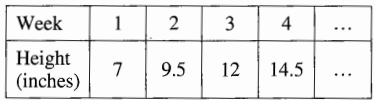# ParaPro Math Practice Test 2023 Questions Answers

ParaPro Math Practice Test 2023 Questions Answers: You can also download a printable PDF. Prepare for the ParaPro Assessment (1755) with ETS test developers’ interactive mathematics practice test.

Directions for Questions 31-60: The questions or incomplete statements below are followed by four suggested answers or completions. Select the best one in each case and fill in the corresponding lettered space on the answer sheet with a heavy, dark mark so that you cannot see the letter.

 Name of the Test ParaPro Assessment Test Administrated by ETS Total Questions 30 Question format multiple-choice questions Test subjects math.

## ParaPro Math Practice Test

Q1. 6,200- 945 =?

• (A) 5,254
• (B) 5,255
• (C) 5,365
• (D) 6,365

Q2. The length of a bulletin board is 5\frac12 feet. What is the length of the bulletin board in inches?

• (A) 55 inches
• (B) 56 inches
• (C) 60 inches
• (D) 66 inches

Q3. Which of the following is a hexagon?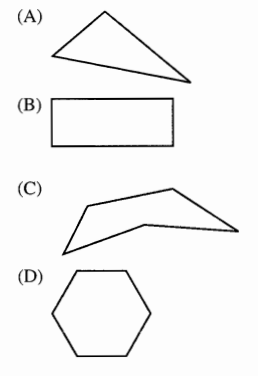Q4. What is the sum of 7 and 5 ?

• (A) 1/2
• (B) 2
• (C) 12
• (D) 35

Q5. Which of the following is equivalent to half a dollar?

• (A) $1.00 • (B)$50
• (C) 0.50¢
• (D) 50 cents

Q6. Which of the following is the closest approximation to 23.62 + 26.81 + 24.12 ?

• (A) 60
• (B) 75
• (C) 90
• (D) 100

Q7. Which of the following numbers is greater than 0.25?

• (A) 0.3
• (B) 0.02
• (C) 0.23
• (D) 0.2

Q8. What value should be placed in the shaded box in the partially completed multiplication table below?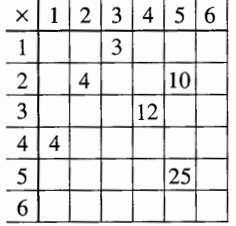• (A) 3
• (B) 8
• (C) 12
• (D) 18

Q9. Which of the following is true?

• (A) 4 < 9
• (B) 4 > 9
• (C) 4 :::: 9
• (D) 4 = 9

Q10. 42.58 + 1.5 =

• (A) 42.73
• (B) 43.08
• (C) 43.63
• (D) 44.08

Q11. For a lesson in measurement, a student is assigned to find the perimeter of Mr. Gray’s rectangular classroom, shown above. Which of the following would correctly determine the perimeter of the classroom?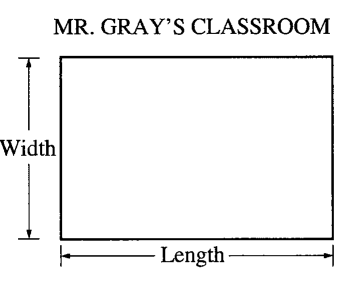• (A) (Length) + (Width)
• (B) (Length) x (Width)
• (C) 2(Length) + 2(Width)
• (D) 2(Length) x 2(Width)

Q12. 34 = ?

• (A) 3 + 4
• (B) 4 + 4 + 4
• (C) 3 X 4
• (D) 3 X 3 X 3 X 3

Q13. On the number line above, where is \frac32  located?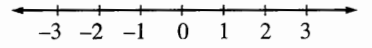• (A) To the left of -1
• (B) Between -1 and 0
• (C) Between 0 and 1
• (D) To the right of 1

Q14. The graph above shows the distribution of musical instruments played by students in a class. If each student plays only one instrument, how many students play the drums? Total number of students in the class = 30• (A) 3
• (B) 7
• (C) 9
• (D) 10

Q15. Mrs. Perez is helping a student solve the word problem shown above. Which of the following is a correct procedure that Mrs. Perez can use?

• (A) 2($0.75) + 4($1.25)
• (B) 4($0.75) + 2($1.25)
• (C) 4($0.75 +$1.25) + 2
• (D) (4 + 2) ($0.75 +$1.25)

Q16. 46. If x + 4 = 14, what is the value of x ?

• (A) 10
• (B) 14
• (C) 18
• (D) 56

Q17. Which of the following numbers has a 2 in the hundredth place?

• (A) 7,430.72
• (B) 2,001.03
• (C) 1,201.46
• (D) 1,007.23

Q18. Which of the following is NOT equivalent to frac12 ?

• (A) \frac{50}{100}
• (B) \frac{2}{8}
• (C) 0.5
• (D) \frac{1.5}{3.0}

Q19. In the xy-plane above, what are the coordinates of point T?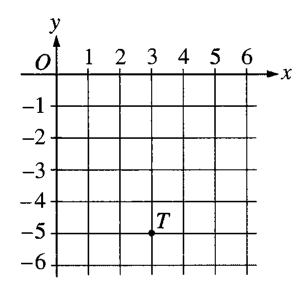• (A) (3, 5)
• (B) (3, 0)
• (C) (3′ -5)
• (D) (-5, 3)

Q20. The total time for 3 class activities is 1\frac14 hour. If the time spent on each activity is the same, how many minutes long is each activity?

• (A) 15 minutes
• (B) 25 minutes
• (C) 30 minutes
• (D) 75 minutes

5, 20, 35, 50, 0 0 0

Q21. In the list above, the first number is 5, and each number after that is 15 more than the previous number. If the pattern is continued, what will be the seventh number on the list?

• (A) 110
• (B) 95
• (C) 85
• (D) 80

Q22. On Tuesday, the school store sold 60 pencils for $0.20 each and 40 pens for$0.50 each. In addition, 8 book bags were sold for $5.00 each. What was the total amount of these sales? • (A)$6.00
• (B) $7.20 • (C)$60.00
• (D) \$72.00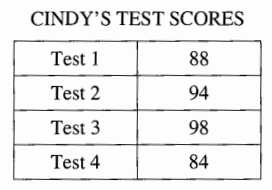Q23. The table above shows Cindy’s test scores on each of the 4 tests. Her final science grade is equal to the average (arithmetic mean) of these scores. What is Cindy’s final science grade?

• (A) 91
• (B) 90
• (C) 88
• (D) 84

Q24. \frac12 + \frac14 = ?

• (A) \frac16
• (B) \frac26
• (C) \frac34
• (D) 1

Q25.

2 X 1 = 2
2×2=2+2
2×3=2+2+2
2×4=2+2+2+2

Which of the following would most likely be the concept that the pattern above is used to teach?

• (A) Subtraction
• (B) Exponents
• (C) Multiplication
• (D) Decimals

Q26.

40 X 600 =?

A student wrote the incorrect number sentence above to calculate 40% of 600. To correct the error, the student’s number sentence should be changed to

• (A) \frac{600}{40} = ?
• (B) \frac{1}{40} × 600 = ?
• (C) \frac{40}{100} × 600 = ?
• (D) \frac{100}{40} × 600 = ?

Q27. What is the approximate value of 20% of 19.89?

• (A) 1.5
• (B) 2
• (C) 3.25
• (D) 4

Q28. 5 + 75 + 25 – 5 =

• (A) 8.75
• (B) 4
• (C) 3
• (D) -1.8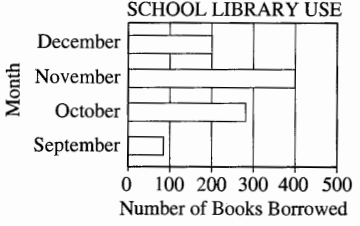Q29. According to the graph above, the total number of books borrowed from the school library for the four months shown is

• (A) less than 900
• (B) between 900 and 1,000
• (C) between 1,000 and 1,100
• (D) greater than 1, 1 00